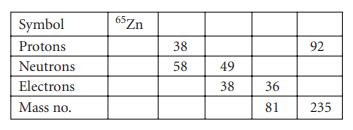×
Get Full Access to Chemistry: The Central Science - 12 Edition - Chapter 2 - Problem 26e
Get Full Access to Chemistry: The Central Science - 12 Edition - Chapter 2 - Problem 26e

×

# Fill in the gaps in the following table, assuming eachISBN: 9780321696724 27

## Solution for problem 26E Chapter 2

Chemistry: The Central Science | 12th Edition

• Textbook Solutions
• 2901 Step-by-step solutions solved by professors and subject experts
• Get 24/7 help from StudySoup virtual teaching assistantsChemistry: The Central Science | 12th Edition

4 5 1 267 Reviews
15
4
Problem 26E

Fill in the gaps in the following table, assuming each column represents a neutral atom.Step-by-Step Solution:
Step 1 of 3

Chapter 4 of “Introductory Chemistry” by Zumdahl and Decoste, 7 /8 edition Law of Constant Composition: a given compound always has the same composition, regardless of where it comes from Ex: carbon dioxide is always 2.7g of oxygen per 1g of carbon Dalton’s Atomic Theory 1. “Elements are made of tiny particles called atoms” 2. “All atoms of a given element are identical” *Later corrected by Dalton to (#2): “All atoms of the same element contain the same number of protons and electrons, but atoms of a given element may have different numbers of neutrons” 3. “The atoms of a given element are different from those of any other element” 4. “Atoms of one element can combine with atoms of other elements to form compounds. A given compound always has the same relative numbers and types of atoms.” 5. “Atoms are indivisible in chemical processes. That is, atoms are not created or destroyed IN CHEMICAL REACTIONS. A chemical reaction simply changes the way the atoms are grouped together.” Compound: distinct substance composed of two or more elements Rules for Writing Formulas 1. Each atom present is represented by its element symbol 2. The number of each type of atom is indicated by a subscript written to the right of the element symbol. 3. When only one atom of a given type is present, the subscript 1 is not written Ex: (one atom of sulfur)  SO 3 (3 atoms of oxygen) Atoms are made up of subatomic parts called electrons as well as protons

Step 2 of 3

Step 3 of 3

## Discover and learn what students are asking

Calculus: Early Transcendental Functions : Basic Differentiation Rules and Rates of Change
?Finding a Value In Exercises 65–70, find k such that the line is tangent to the graph of the function. Function

Calculus: Early Transcendental Functions : Inverse Trigonometric Functions: Integration
?In Exercises 1-20, find the indefinite integral. $$\int \frac{1}{x \sqrt{4 x^{2}-1}} d x$$

Calculus: Early Transcendental Functions : Iterated Integrals and Area in the Plane
?In Exercises 1 - 10, evaluate the integral. $$\int_{x^{3}}^{\sqrt{x}}\left(x^{2}+3 y^{2}\right) d y$$

Chemistry: The Central Science : Molecular Geometry and Bonding Theories
?What is the distinction between a bond dipole and a molecular dipole moment?

Chemistry: The Central Science : Gases
?Complete the following table for an ideal gas:

Unlock Textbook Solution